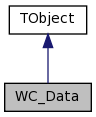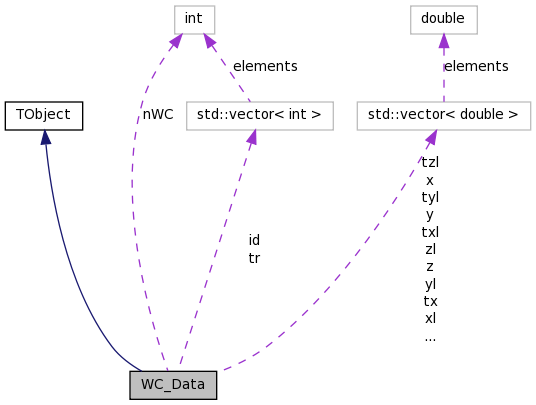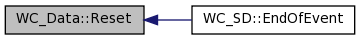WC_Data Class Reference

WC_Data class. More...

`#include <WC_Data.h>`

Inheritance diagram for WC_Data:[legend]
Collaboration diagram for WC_Data:[legend]

List of all members.

Public Member Functions

WC_Data ()
Constructor.
~WC_Data ()
Destructor.
void Reset ()
Clear the event vectors.

Private Attributes

vector< double > e
vector< int > id
int nWC
vector< double > t
vector< int > tr
vector< double > tx
vector< double > txl
vector< double > ty
vector< double > tyl
vector< double > tz
vector< double > tzl
vector< double > x
vector< double > xl
vector< double > y
vector< double > yl
vector< double > z
vector< double > zl

class WC_SD

Detailed Description

WC_Data class.

Defines the class used for writing data to the data file in the WC TBranch.

Definition at line 36 of file WC_Data.h.

Constructor & Destructor Documentation

 WC_Data::WC_Data ( )

Constructor.

 WC_Data::~WC_Data ( )

Destructor.

Definition at line 36 of file WC_Data.cc.

Member Function Documentation

 void WC_Data::Reset ( )

Clear the event vectors.

Definition at line 42 of file WC_Data.cc.

Referenced by WC_SD::EndOfEvent().

Here is the caller graph for this function:Friends And Related Function Documentation

 friend class WC_SD` [friend]`

Definition at line 56 of file WC_Data.h.

Member Data Documentation

 vector WC_Data::e` [private]`

Definition at line 63 of file WC_Data.h.

Referenced by WC_SD::EndOfEvent().

 vector WC_Data::id` [private]`

Definition at line 60 of file WC_Data.h.

Referenced by WC_SD::EndOfEvent().

 int WC_Data::nWC` [private]`

Definition at line 58 of file WC_Data.h.

Referenced by WC_SD::EndOfEvent().

 vector WC_Data::t` [private]`

Definition at line 64 of file WC_Data.h.

Referenced by WC_SD::EndOfEvent().

 vector WC_Data::tr` [private]`

Definition at line 61 of file WC_Data.h.

Referenced by WC_SD::EndOfEvent().

 vector WC_Data::tx` [private]`

Definition at line 66 of file WC_Data.h.

Referenced by WC_SD::EndOfEvent().

 vector WC_Data::txl` [private]`

Definition at line 70 of file WC_Data.h.

Referenced by WC_SD::EndOfEvent().

 vector WC_Data::ty` [private]`

Definition at line 67 of file WC_Data.h.

Referenced by WC_SD::EndOfEvent().

 vector WC_Data::tyl` [private]`

Definition at line 71 of file WC_Data.h.

Referenced by WC_SD::EndOfEvent().

 vector WC_Data::tz` [private]`

Definition at line 68 of file WC_Data.h.

Referenced by WC_SD::EndOfEvent().

 vector WC_Data::tzl` [private]`

Definition at line 72 of file WC_Data.h.

Referenced by WC_SD::EndOfEvent().

 vector WC_Data::x` [private]`

Definition at line 74 of file WC_Data.h.

Referenced by WC_SD::EndOfEvent().

 vector WC_Data::xl` [private]`

Definition at line 78 of file WC_Data.h.

Referenced by WC_SD::EndOfEvent().

 vector WC_Data::y` [private]`

Definition at line 75 of file WC_Data.h.

Referenced by WC_SD::EndOfEvent().

 vector WC_Data::yl` [private]`

Definition at line 79 of file WC_Data.h.

Referenced by WC_SD::EndOfEvent().

 vector WC_Data::z` [private]`

Definition at line 76 of file WC_Data.h.

Referenced by WC_SD::EndOfEvent().

 vector WC_Data::zl` [private]`

Definition at line 80 of file WC_Data.h.

Referenced by WC_SD::EndOfEvent().

The documentation for this class was generated from the following files: